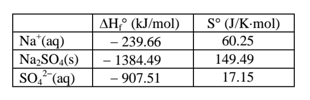# Problem: A. Using the thermodynamic data provided for dissolving Na 2SO4(s) in water, that is, for the chemical process Na2SO4(s) → 2Na+(aq) + SO42-(aq)         Calculate ΔH°.   B. When I calculated ΔS°, I got -11.84 J/K•mol. Using this value and your answer to part A, calculate ΔG° at 25°C.     C. Which term is responsible for the temperature dependence of ΔG: enthalpy (ΔH°), entropy (ΔS°), both, or neither (ΔG is not temperature dependent)? Will increasing the temperature make ΔG larger (more positive), smaller (more negative), or have no effect? D. So based on your answer to part C, will the solubility of Na 2SO4 increase or decrease with increasing temperature?

###### FREE Expert Solution
81% (181 ratings)
###### Problem Details

A. Using the thermodynamic data provided for dissolving Na 2SO4(s) in water, that is, for the chemical process

Na2SO4(s) → 2Na+(aq) + SO42-(aq)         Calculate ΔH°.

B. When I calculated ΔS°, I got -11.84 J/K•mol. Using this value and your answer to part A, calculate ΔG° at 25°C.

C. Which term is responsible for the temperature dependence of ΔG: enthalpy (ΔH°), entropy (ΔS°), both, or neither (ΔG is not temperature dependent)?

Will increasing the temperature make ΔG larger (more positive), smaller (more negative), or have no effect?

D. So based on your answer to part C, will the solubility of Na 2SO4 increase or decrease with increasing temperature?What scientific concept do you need to know in order to solve this problem?

Our tutors have indicated that to solve this problem you will need to apply the Gibbs Free Energy concept. You can view video lessons to learn Gibbs Free Energy. Or if you need more Gibbs Free Energy practice, you can also practice Gibbs Free Energy practice problems.

What is the difficulty of this problem?

Our tutors rated the difficulty ofA. Using the thermodynamic data provided for dissolving Na 2...as high difficulty.

How long does this problem take to solve?

Our expert Chemistry tutor, Dasha took 12 minutes and 22 seconds to solve this problem. You can follow their steps in the video explanation above.

What professor is this problem relevant for?

Based on our data, we think this problem is relevant for Professor Evans' class at QC CUNY .